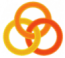General
 Course Home Page ESSLLI 2004Material
Lecture 1
 Slides Practical Session
Lecture 2
 Slides Practical Session
Lecture 3
 Slides Practical Session
Lecture 4
 Slides Practical Session
Lecture 5
 Slides Practical Session

# Operations on vectors

In mathematics, an n-dimensional vector is a list of numbers of length n. For example, `[2,5,12]` is a 3-dimensional vector, and `[45,27,3,-4,6]` is a 5-dimensional vector. One of the basic operations on vectors is scalar multiplication. In this operation, every element of a vector is multiplied by some number. For example, if we scalar multiply the 3-dimensional vector `[2,7,4]` by 3 the result is the 3-dimensional vector `[6,21,12]`. Write a 3-place predicate `scalarMult` whose first argument is an integer, whose second argument is a list of integers, and whose third argument is the result of scalar multiplying the second argument by the first. For example, the query `scalarMult(3,[2,7,4],Result)` should yield `Result = [6,21,12].`
Hint

Another fundamental operation on vectors is the dot product. This operation combines two vectors of the same dimension and yields a number as a result. The operation is carried out as follows: the corresponding elements of the two vectors are multiplied, and the results added. For example, the dot product of `[2,5,6]` and `[3,4,1]` is 6+20+6, that is, 32. Write a 3-place predicate dot whose first argument is a list of integers, whose second argument is a list of integers of the same length as the first, and whose third argument is the dot product of the first argument with the second. For example, the query `dot([2,5,6],[3,4,1],Result)` should yield `Result = 32`.
Hint